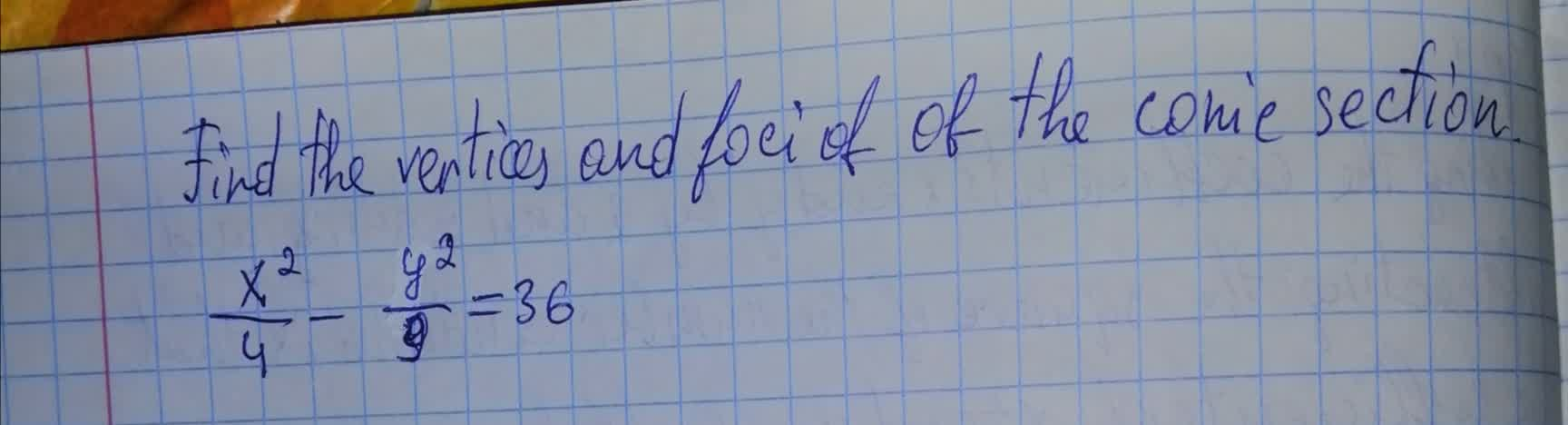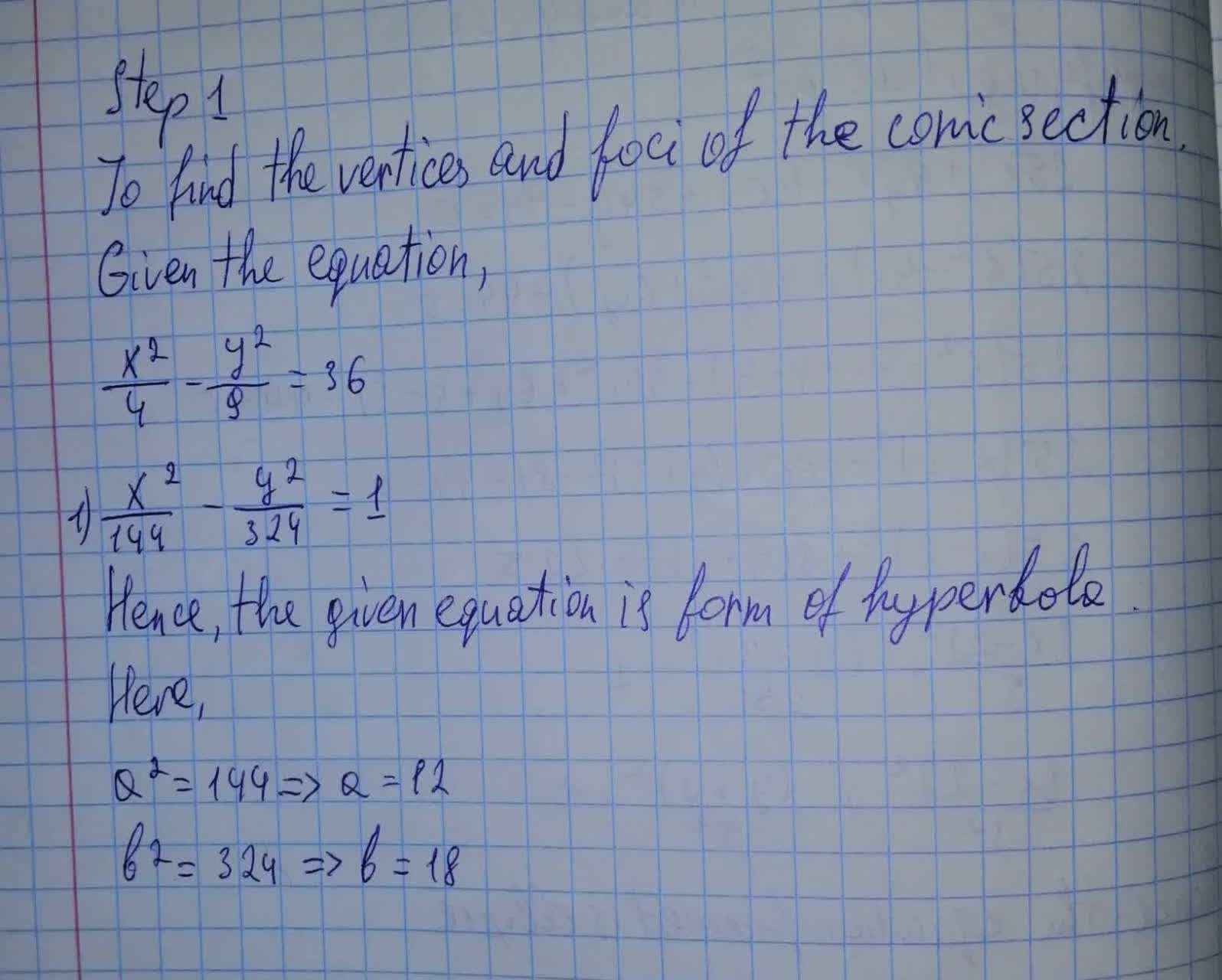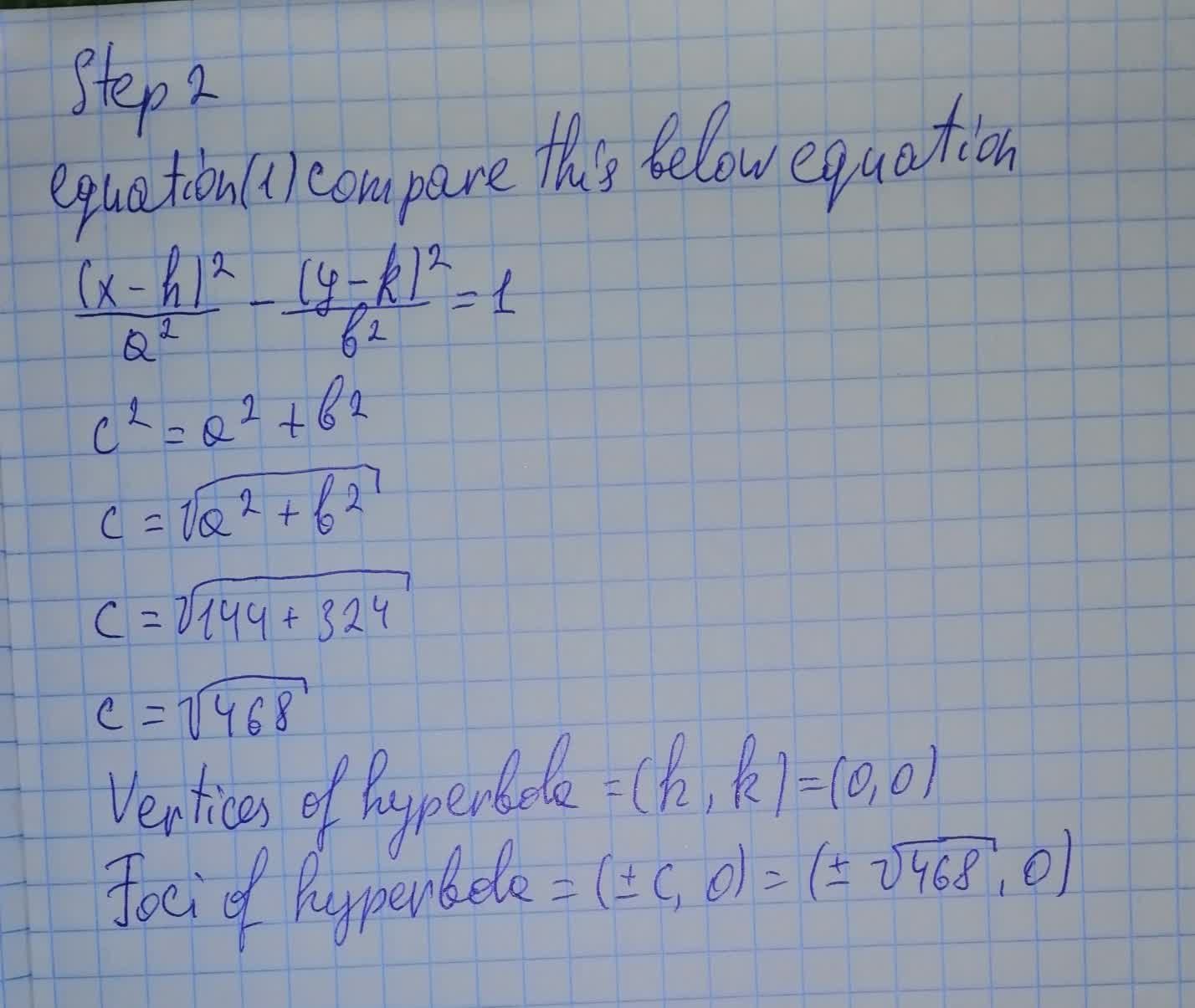# Find the vertices and foci of the conic section. x^2/4 − y^2/9 = 36tabita57i 2021-08-11 Answered

Find the vertices and foci of the conic section. $\frac{{x}^{2}}{4}-\frac{{y}^{2}}{9}=36$You can still ask an expert for help

• Questions are typically answered in as fast as 30 minutes

Solve your problem for the price of one coffee

• Math expert for every subject
• Pay only if we can solve itMaciej Morrow

The conic section has solution:###### Not exactly what you’re looking for?Vasquez

Step 1

To find the vertices and foci of the conic section.

Given the equation,

$\frac{{x}^{2}}{4}-\frac{{y}^{2}}{9}=36$

1)\$\frac{{x}^{2}}{144}-\frac{{y}^{2}}{324}=1$

Hence, the given equation is form of hyperbola.

Here,

${a}^{2}=144⇒a=12$

${b}^{2}=324⇒b=18$

Step 2

equation (1) compare this below equation

$\frac{\left(x-h{\right)}^{2}}{{a}^{2}}-\frac{\left(y-k{\right)}^{2}}{{b}^{2}}=1$

${c}^{2}={a}^{2}+{b}^{2}$

$c=\sqrt{{a}^{2}+{b}^{2}}$

$c=\sqrt{144+324}$

$c=\sqrt{468}$

Vertices of hyperbola $=\left(h,k\right)=\left(0,0\right)$

Foci of hyperbola $=\left(±c,0\right)=\left(±\sqrt{468},0\right)$